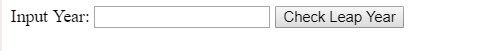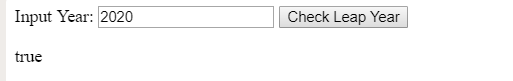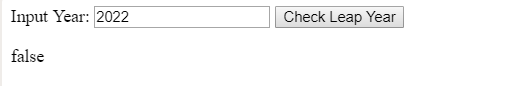Open in App
Not now

# JavaScript program to check if a given year is leap year

• Difficulty Level : Medium
• Last Updated : 12 Apr, 2019

Prerequisite:Understanding basic JavaScript codes.
A year is leap year if following conditions are satisfied:

• Year is multiple of 400.
• Year is multiple of 4 and not multiple of 100.

Approach:

• Get the value of input field by using document.getElementById(“year”).value
• Check the given year is leap year or not by using JavaScript expression and display its value.

Example:

 `````<``html``>`` ` `<``head``>``    ``<``title``>``        ``JavaScript to check leap year``    `````` ` `<``body``>``    ``Input Year: <``input` `type``=``"text"` `id` `= ``"year"``/>``     ` `    ``<``input` `type``=``"button"` `id``=``"button"` `onClick``=``"isLeapYear()"``            ``value``=``"Check Leap Year"``>``     ` `    ``<``p` `id``=``"GFG"``>``     ` `    ````    ``<``script``>``        ``function isLeapYear() {``            ``var year= document.getElementById("year").value;``             ` `            ``document.getElementById("GFG").innerHTML ``                ``= (year % 100 === 0) ? (year % 400 === 0)``                                     ``: (year % 4 === 0);``        ``}``    `````` ` `                    `

Output:

• Before input year:• After input leap year:• After input not leap year:My Personal Notes arrow_drop_up